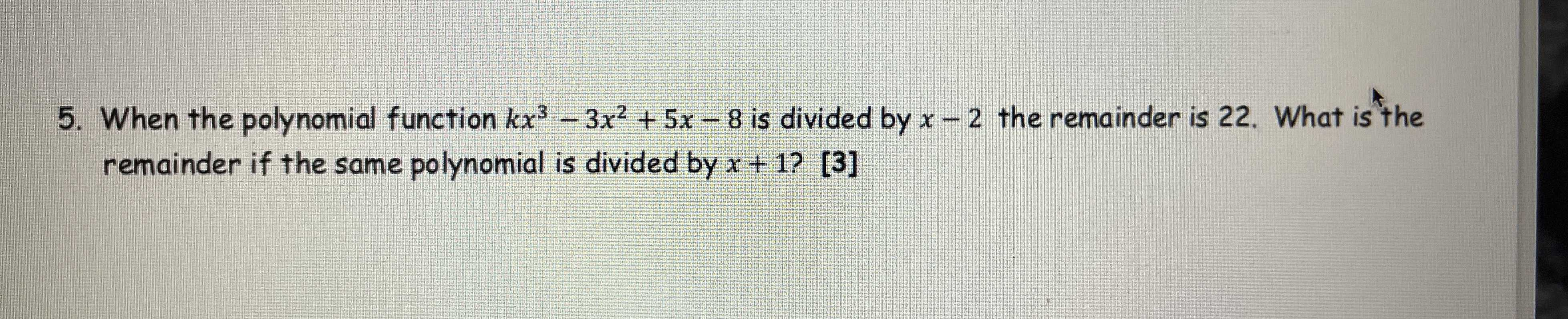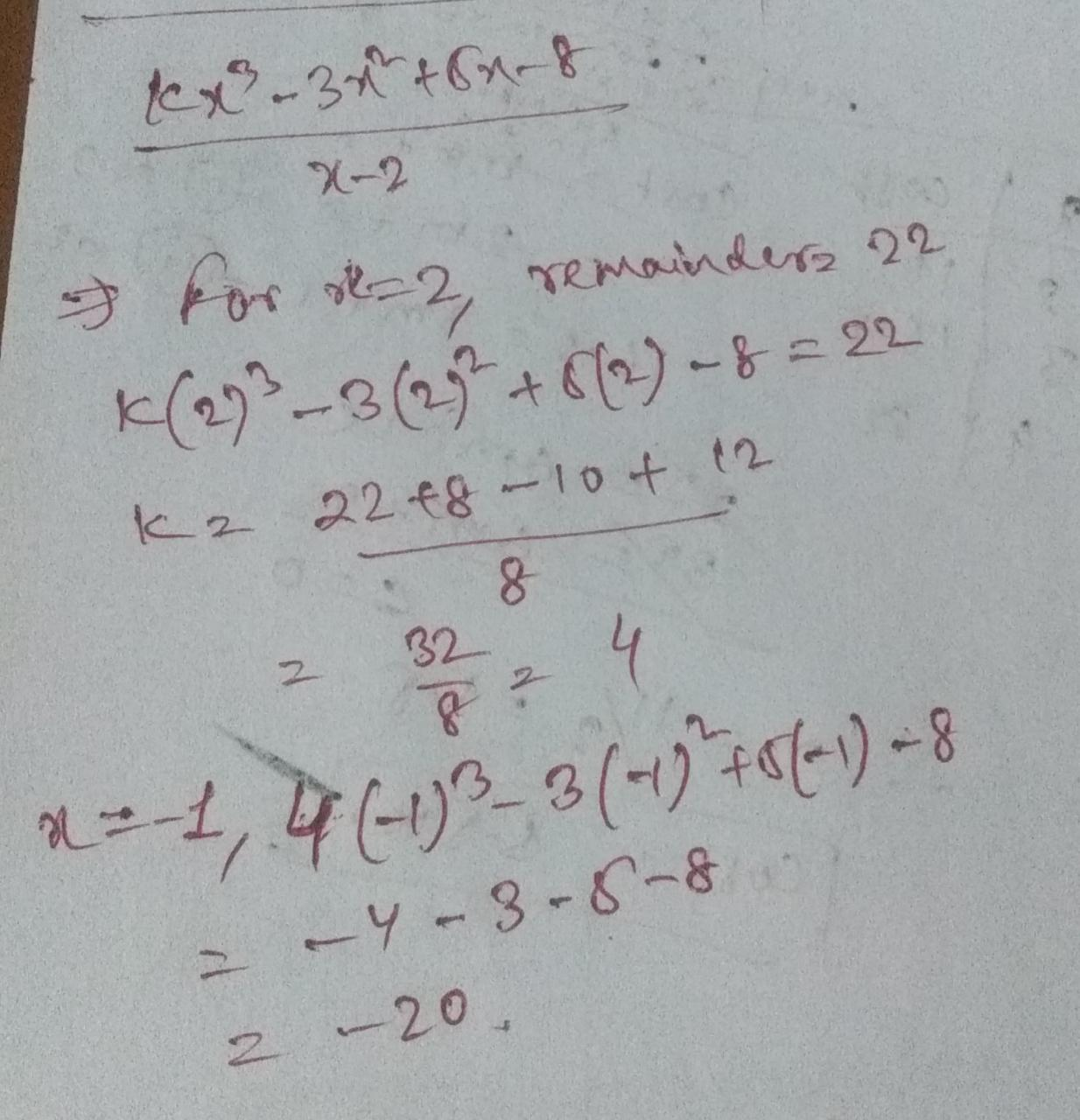### ¿Todavía tienes preguntas de matemáticas?

Pregunte a nuestros tutores expertos
Algebra
Pregunta5. When the polynomial function $$k x ^ { 3 } - 3 x ^ { 2 } + 5 x - 8$$ is divided by $$x - 2$$ the remainder is $$22$$ . What is the remainder if the same polynomial is divided by $$x + 1$$ ?